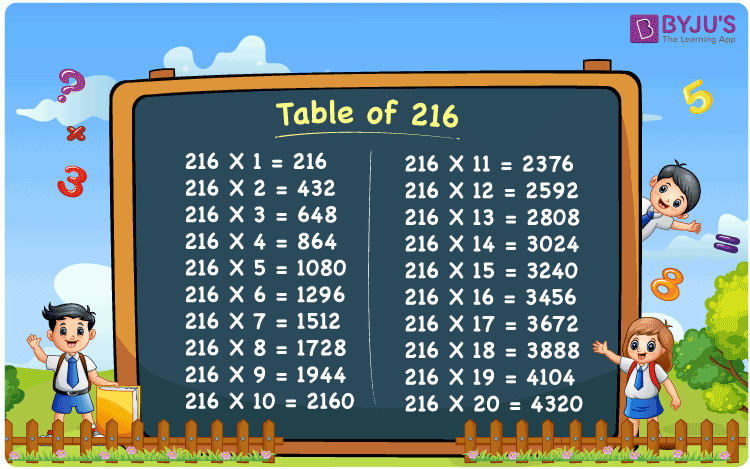Checkout JEE MAINS 2022 Question Paper Analysis : Checkout JEE MAINS 2022 Question Paper Analysis :

# Table of 216

Table of 216 is the multiplication table that represents the values produced when 208 is multiplied by natural numbers. These values are also called multiples of 216. We can also express 216 times table as the repeated addition of the original number. For example, 216 x 3 = 216 + 216 + 216 = 648. In this article, we have provided a table of two hundred and sixteen up to 20 times. Learn more about multiplication tables and download the PDF of table 216 from below.

## Table of 216 Chart## What is 216 Times Table?

Learn how to write the 216 times table, with the help of the repeated addition table given below.

 216×1 = 216 216 216×2 = 432 216 + 216 = 432 216×3 = 648 216 + 216 + 216 = 648 216×4 = 864 216 + 216 + 216 + 216 = 864 216×5 = 1080 216 + 216 + 216 + 216 + 216 = 1080 216×6 = 1296 216 + 216 + 216 + 216 + 216 + 216 = 1296 216×7 = 1512 216 + 216 + 216 + 216 + 216 + 216 + 216 = 1512 216×8 = 1728 216 + 216 + 216 + 216 + 216 + 216 + 216 + 216 = 1728 216×9 = 1944 216 + 216 + 216 + 216 + 216 + 216 + 216 + 216 + 216 = 1944 216×10 = 2160 216 + 216 + 216 + 216 + 216 + 216 + 216 + 216 + 216 + 216 = 2160

## Multiplication table of 216

Here is the table of 216 up to 20 times below.

 216 × 1 = 216 216 × 2 = 432 216 × 3 = 648 216 × 4 = 864 216 × 5 = 1080 216 × 6 = 1296 216 × 7 = 1512 216 × 8 = 1728 216 × 9 = 1944 216 × 10 = 2160 216 × 11 = 2376 216 × 12 = 2592 216 × 13 = 2808 216 × 14 = 3024 216 × 15 = 3240 216 × 16 = 3456 216 × 17 = 3672 216 × 18 = 3888 216 × 19 = 4104 216 × 20 = 4320

## Solved Examples on Table of 216

Q.1: Evaluate the value of 1000 – 216 x 4.

Solution: Given,

⇒ 1000 – 216 x 4

⇒ 1000 – 864

⇒ 136

Q.2: What is the value of 216 multiplied by 5 plus 250?

Solution: Given,

216 multiplied by 5 plus 250

⇒ 216 x 5 + 250

⇒ 1080 + 250

⇒ 1330

## Frequently Asked Questions on Table of 216

### What is table of 216?

Table of 216 is the multiplication table that represents the repeated addition of 216, when it is multiplied by any number.

### How to read 216 times table?

Table of 216 can be read as:

Two Hundred And Sixteen times One is equal to Two Hundred And Sixteen

Two Hundred And Sixteen times Two is equal to Four Hundred And Thirty-Two

Two Hundred And Sixteen times Three is equal to Six Hundred And Forty-Eight

Two Hundred And Sixteen times Four is equal to Eight Hundred And Sixty-Four

And so on.

### What is the value of 216 times 11?

The value of 216 times 11 is equal to 2376.

### What should be multiplied by 216 to get 1728?

From the table of 216, when 216 is multiplied by 8 the value is 1728.